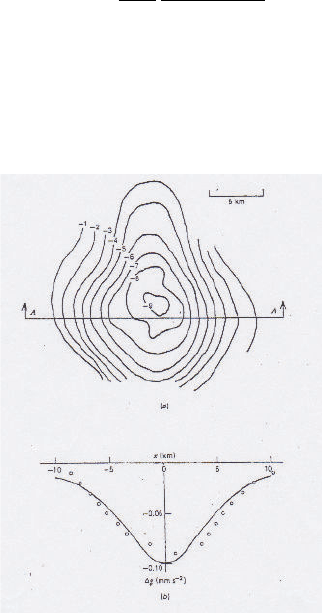Study Guides (400,000)
US (230,000)
UCSB (3,000)
EARTH (10)
Final

# EARTH 135 Lecture Notes - Lecture 15: Salt Dome, Gravity Anomaly, Gravitational AccelerationExam

Department
Earth Science
Course Code
EARTH 135
Professor
Tanimoto
Study Guide
Final

This preview shows pages 1-2. to view the full 6 pages of the document.Earth 135 : Gravity Anomaly (May 27, 2020)
Read 5.6, 5.7, 5.8, 5.11 in Turcotte and Schubert.
Modeling
Buried uniform sphere
A low-density salt dome (ρ= 2150 kg/m3) exists in higher density carbonate rocks (ρ=
2500 kg/m3). Density diﬀerence is ∆ρ=350 kg/m3. Assume this salt dome can be approxi-
mated by a sphere of radius Rwith its center at depth zbelow the surface.
What is the gravity anomaly caused by this salt dome ? Show that it becomes
gz=4πG
3
ρR3z
(z2+x2)3/2(1)
Note that gravitational acceleration gis measured vertically downward, not toward the anomaly, as
we usually don’t know the location of such a salt dome.
Given the data in Figure 1, what is your estimate of Rand z?
Figure 1: Gravity anomaly due to a buried spherical body
1

Only pages 1-2 are available for preview. Some parts have been intentionally blurred.Horizontal cylinder
Let us assume an inﬁnite cylinder with radius Ris lying at depth z. This can be a model for an
anticline or a syncline in geology, but here we consider it to be a subway tunnel. Show that gravity
anomaly at x on the surface (z= 0) can be written
gz= 2πGρR2
z
1
1 + (x/z)2(2)
Note that xis the distance on the surface from the surface projection of the cylinder, zis the
depth to the tunnel (the center of the tunnel), and the extent of the tunnel is −∞ < y < .
Gravitational acceleration ∆gzat x and z= 0 in the ﬁgure can be written (the depth to the
tunnel is z)
gz=Z
−∞
G(πR2ρ)
x2+z2+y2
z
px2+z2+y2dy
where the term just in front of dy is the projection of gonto the vertical direction. If you can show
Z
−∞
dy
(x2+z2+y2)3/2=2
x2+z2
you have the proof.
Figure 2: Plan view (from above) of a buried tunnel in the y direction
Question 1: Suppose there is an underground tunnel of a circular cross section with radius 5 m.
The medium surrounding it has density 2800 kg/m3. If the axis of the tunnel lies 50 m below the
surface, what is the gravity anomaly due to this tunnel ?
Question 2: Solve Problem 5.12 in Turcotte and Schubert (p245). Type in the MATLAB problem
in Appendix D of this textbook and get your solution.
2